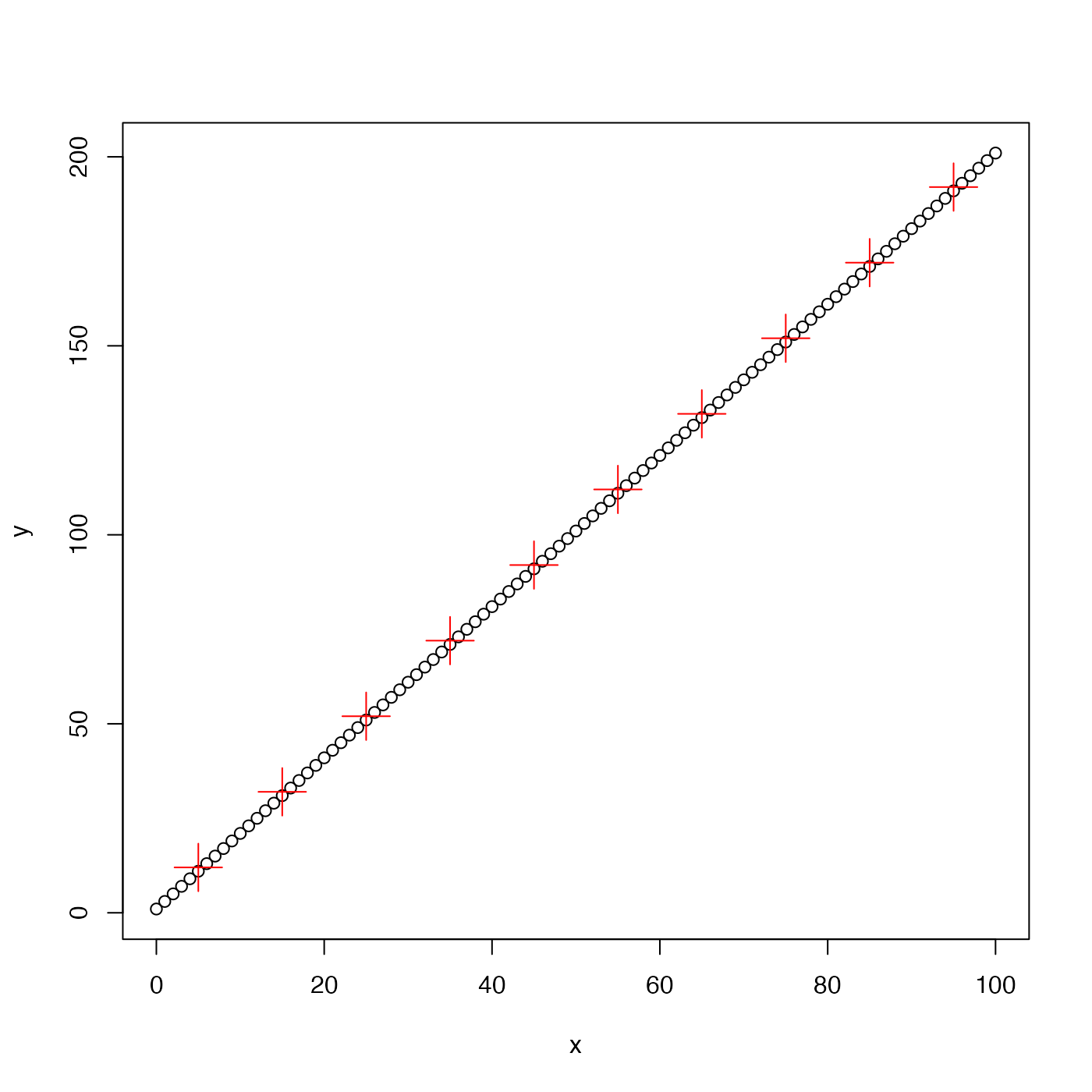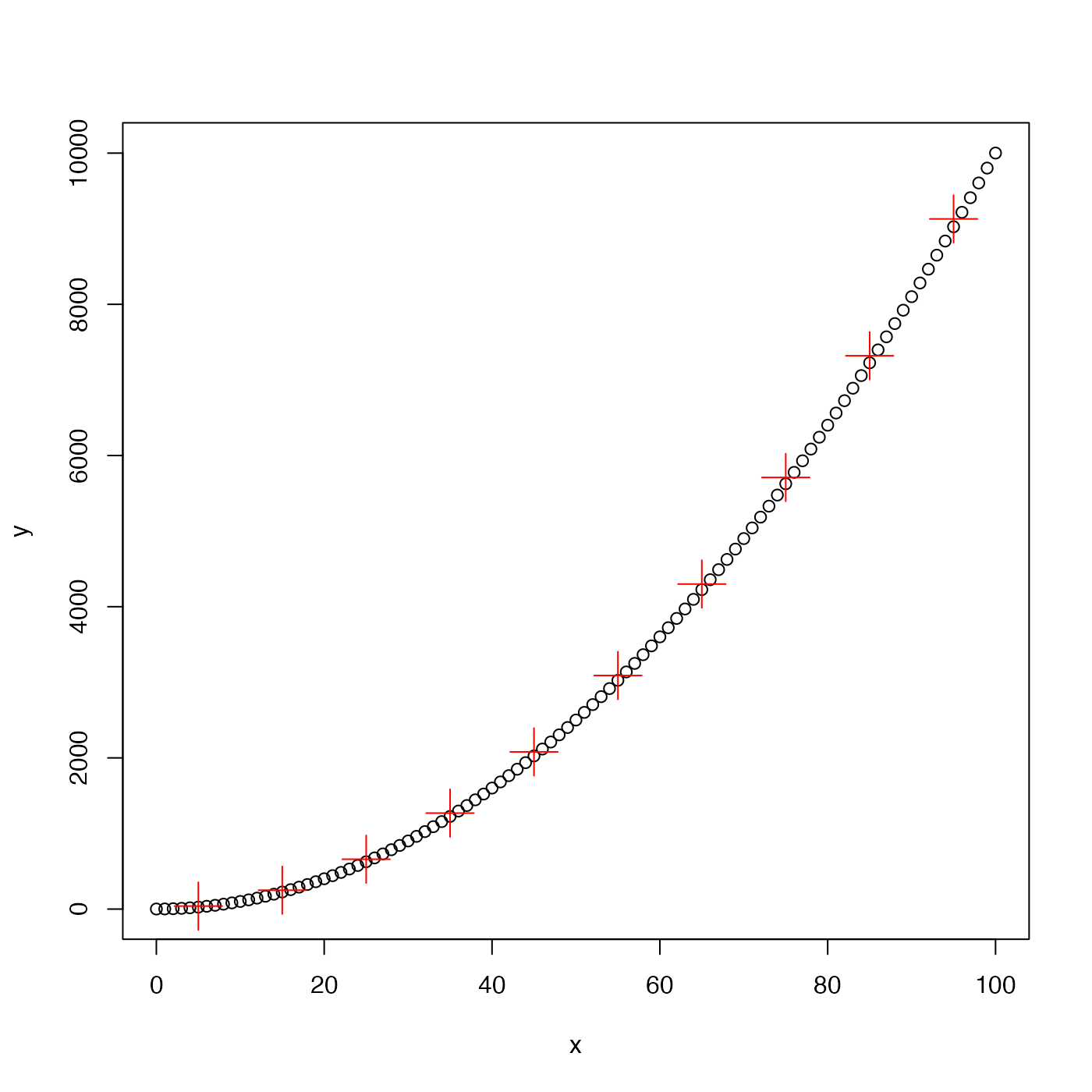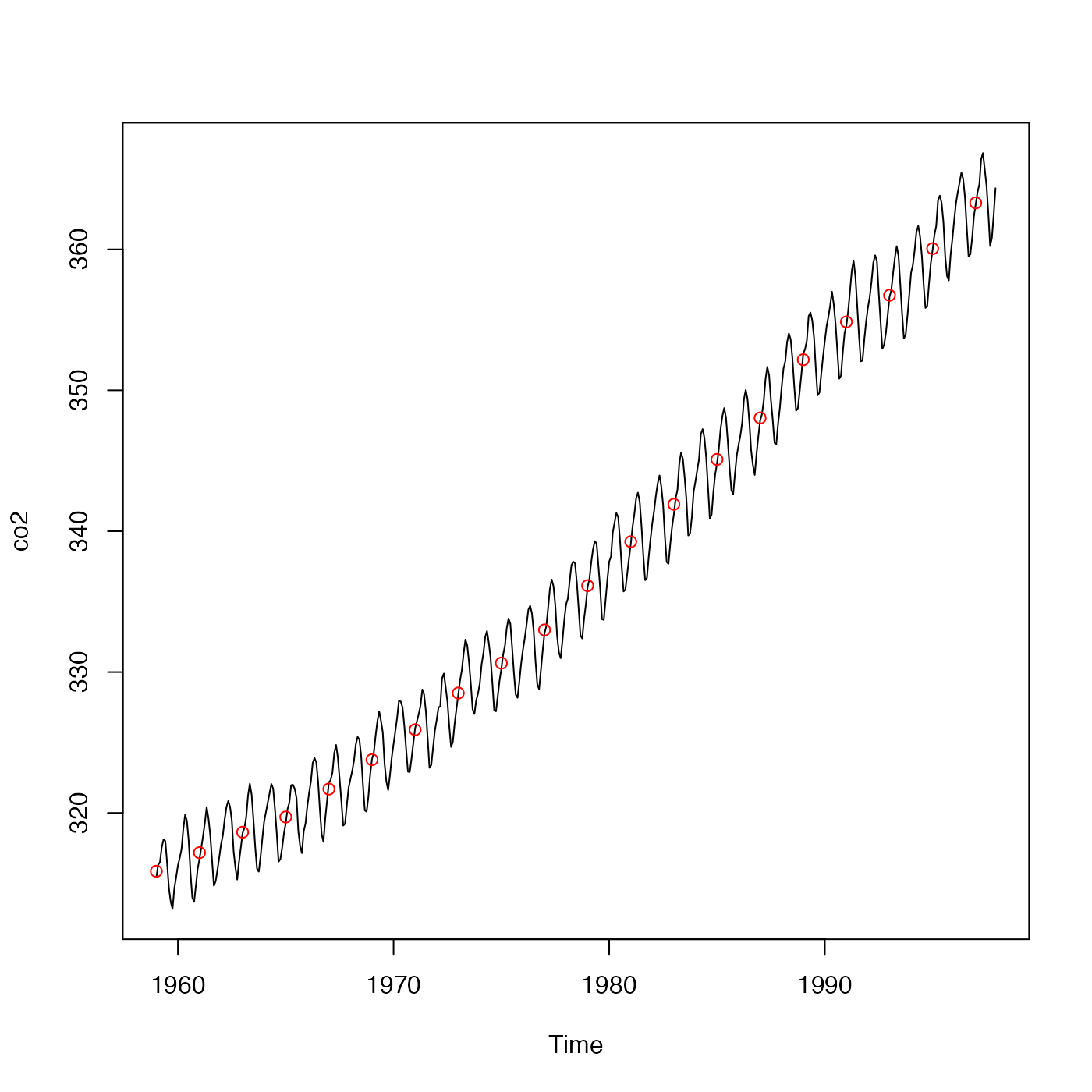The y vector is averaged in bins defined for x. Missing values in y are ignored.

binAverage(x, y, xmin, xmax, xinc)

## Arguments

x a vector of numerical values. a vector of numerical values. x value at the lower limit of first bin; the minimum x will be used if this is not provided. x value at the upper limit of last bin; the maximum x will be used if this is not provided. width of bins, in terms of x value; 1/10th of xmax-xmin will be used if this is not provided.

## Value

A list with two elements: x, the mid-points of the bins, and y, the average y value in the bins.

Other bin-related functions: binApply1D(), binApply2D(), binCount1D(), binCount2D(), binMean1D(), binMean2D()

## Examples

library(oce)
## A. fake linear data
x <- seq(0, 100, 1)
y <- 1 + 2 * x
plot(x, y, pch=1)ba <- binAverage(x, y)
points(ba$x, ba$y, pch=3, col='red', cex=3)points(ba$x, ba$y, pch=3, col='red', cex=3)points(avg$x, avg$y, col='red')